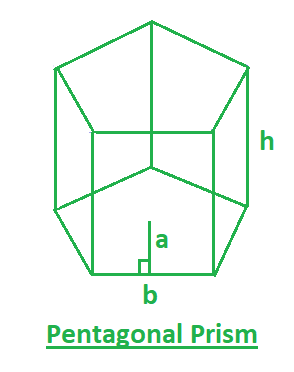Open in App
Not now

# Program to find volume and surface area of pentagonal prism

• Last Updated : 28 Jun, 2022

A prism that has 5 rectangular faces and 2 parallel pentagonal bases is a pentagonal prism. So you are given the apothem length(a), base length(b) and height(h) of the pentagonal prism. you have to find the surface area and the volume of the Pentagonal Prism.
Examples:

Input : a=3, b=5, h=6
Output :surface area=225, volume=225

Input : a=2, b=3, h=5
Output :surface area=105, volume=75In this figure,
a– apothem length of the Pentagonal Prism.
b– base length of the Pentagonal Prism.
h– height of the Pentagonal Prism.
Formulas:Below are the formulas for calculating the surface area and the volume of the Pentagonal Prism.## C++

 // CPP program to find // surface area and volume of the // Pentagonal Prism #include  using namespace std;   // function for surface area float surfaceArea(float a, float b, float h) {     return 5 * a * b + 5 * b * h; }   // function for VOlume float volume(float b, float h) {     return (5 * b * h) / 2; }   // Driver function int main() {     float a = 5;     float b = 3;     float h = 7;       cout << "surface area= " << surfaceArea(a, b, h) << ", ";       cout << "volume= " << volume(b, h); }

## Java

 // Java program to find // surface area and volume of the // Pentagonal Prism import java.util.*;   class solution {   // function for surface area static float surfaceArea(float a, float b, float h) {       return 5 * a * b + 5 * b * h;   }   // function for VOlume static float volume(float b, float h) {       return (5 * b * h) / 2;   }   // Driver function public static void main(String arr[]) {     float a = 5;     float b = 3;     float h = 7;       System.out.println( "surface area= "+surfaceArea(a, b, h)+", ");       System.out.println("volume= "+volume(b, h)); } }

## Python3

 # Python 3 program to find surface area # and volume of the Pentagonal Prism   # function for surface area def surfaceArea(a, b, h):     return 5 * a * b + 5 * b * h   # function for VOlume def volume(b, h):     return (5 * b * h) / 2   # Driver Code if __name__ == '__main__':     a = 5     b = 3     h = 7       print("surface area =", surfaceArea(a, b, h),                    ",", "volume =", volume(b, h))   # This code is contributed by # Sanjit_Prasad

## C#

 // C# program to find surface // area and volume of the // Pentagonal Prism using System;   class GFG {   // function for surface area static float surfaceArea(float a,                           float b, float h) {     return 5 * a * b + 5 * b * h; }   // function for VOlume static float volume(float b, float h) {     return (5 * b * h) / 2; }   // Driver Code public static void Main() {     float a = 5;     float b = 3;     float h = 7;       Console.WriteLine("surface area = " +              surfaceArea(a, b, h) + ", ");       Console.WriteLine("volume = " +                      volume(b, h)); } }   // This code is contributed by vt_m

## PHP

 

## Javascript

 

Output:

surface area= 180, volume= 52.5

Time complexity: O(1)

Auxiliary Space: O(1)

My Personal Notes arrow_drop_up
Related Articles